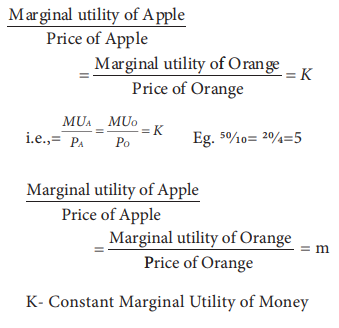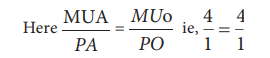Home | | Economics 11th std | The Law of Equi-Marginal Utility

# The Law of Equi-Marginal Utility

The law of diminishing marginal utility is applicable only to the want of a single commodity. B

The Law of Equi-Marginal Utility

The law of diminishing marginal utility is applicable only to the want of a single commodity. But in reality, wants are unlimited and these wants are to be satisfied. Hence, to analyze such a situation, the law of diminishing marginal utility is extended and is called “Law of Equi-Marginal Utility ”. It is also called the “Law of Substitution”, “The Law of Consumers Equilibrium”, “Gossen Second Law” and “The Law of Maximum Satisfaction”.

## Definition

Marshall states the law as, “If a person has a thing which he can put to several uses, he will distribute it among these uses in such a way that it has the same marginal utility in all. For, if it had a greater marginal utility in one use than another he would gain by taking away some of it from the second use and applying it to first”.

## Assumptions

1.        The consumer is rational in the sense that he wants to get maximum satisfaction.

2.        The utility of each commodity is measurable in cardinal numbers.

3.        The marginal utility of money remains constant.

4.        The income of the consumer is given.

5.        There is perfect competition in the market

6.        The prices of the commodities are given.

7.        The law of diminishing marginal utility operates.

## Explanation

The law can be explained with the help of an example. Suppose a consumer wants to spend his limited income on Apple and Orange. He is said to be in equilibrium, only when he gets maximum satisfaction with his limited income. Therefore, he will be in equilibrium, when,In views of this equilibrium, this Law is also called the “Law of Consumers Equilibrium”.In case MUA/PA is less than MUo/PO he would transfer the money from Apple to Orange till it is equal. This process of substitution gives him maximum satisfaction both from Apple and Orange. Hence, this Law is also called “Law of Substitution”. Eg. For Apple 50/25; for Orange 20/4. In such situation, spending more money on orange is wiser.

## Illustration

This Law can be illustrated with the help of table 2.2. Let us assume that the consumer has a given income of Rs. 11. He wants to spend this entire income (i.e., Rs.11) on Apple and Orange. The price of an Apple and the price of an Orange is  Rs.1 each.

If the consumer wants to attain maximum utility, he should buy 6 units of apples and 5 units of oranges, so that he can get (92+58) 150 units. No other combination of Apple and Orange can give higher than 150 utilities.A 2 A3 and B2 B3 lines have not been used for explanation.

## Diagrammatic Illustration

In diagram 2.3, X axis represents the amount of money spent and Y axis represents the marginal utilities of Apple and Orange respectively. If the consumer spends  Rs.6 on

Apple and  Rs.5 on Orange, the marginal utilities of both are equal i.e.,AA1=BB1 (4=4).

Hence, he gets maximum utility.

## Criticisms

1.        In practice, utility cannot be measured, only be felt.

2.        This Law cannot be applied to durable goods.

Tags : Economics , 11th Economics : Chapter 2 : Consumption Analysis
Study Material, Lecturing Notes, Assignment, Reference, Wiki description explanation, brief detail
11th Economics : Chapter 2 : Consumption Analysis : The Law of Equi-Marginal Utility | Economics